# ECE 697 B 667 Spring 2006 Synthesis and

• Slides: 48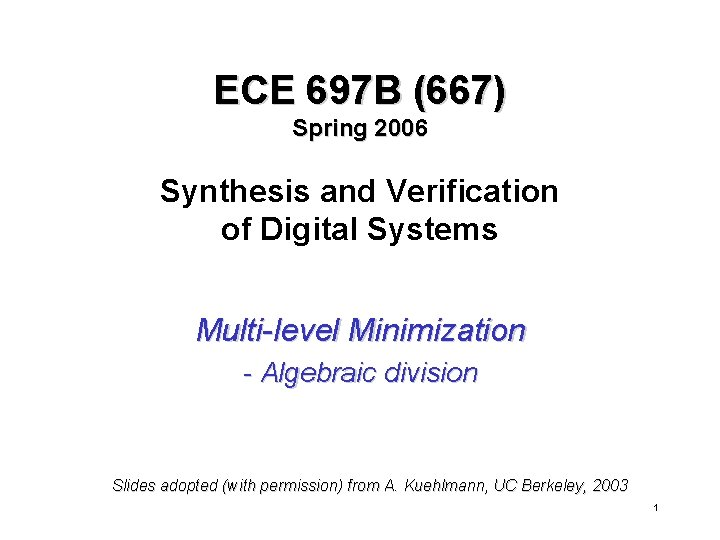ECE 697 B (667) Spring 2006 Synthesis and Verification of Digital Systems Multi-level Minimization - Algebraic division Slides adopted (with permission) from A. Kuehlmann, UC Berkeley, 2003 1Outline • Division and factorization – Definition – Examples • Algebraic vs Boolean division – Algorithms – Applications • Finding good divisors – Kernels and co-kernels ECE 667 - Synthesis & Verification - Lecture 9 2Factorization • Given an F in SOP form, how do we generate a “good” factored form • Division operation: – central in many operations – need to find a good divisor D – apply the actual division • results in quotient Q and remainder R • Applications: – factoring – substitution – extraction ECE 667 - Synthesis & Verification - Lecture 9 3Division • Definition 1: An operation OP is called division if, given two SOP expressions F and G, it generates expressions H and R, such that: F = GH + R – G is called the divisor – H is called the quotient – R is called the remainder • Definition 2: If GH is an algebraic product, then OP is called an algebraic division (denoted F // G) otherwise GH is a Boolean product and OP is a Boolean division (denoted F G). ECE 667 - Synthesis & Verification - Lecture 9 4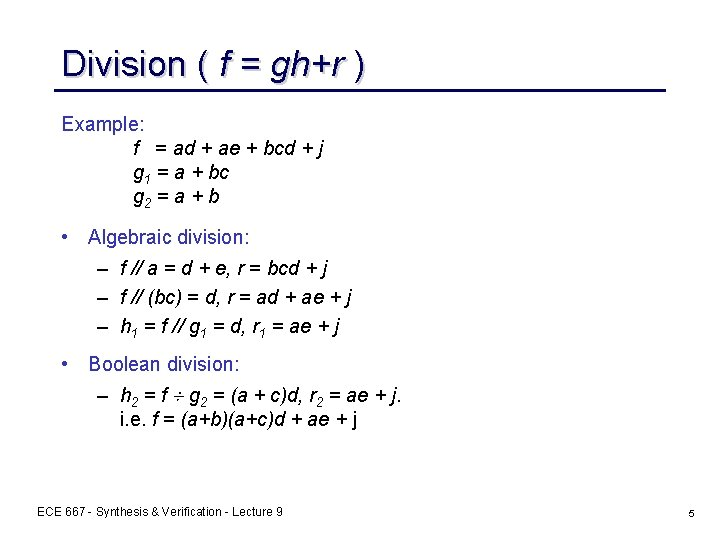Division ( f = gh+r ) Example: f = ad + ae + bcd + j g 1 = a + bc g 2 = a + b • Algebraic division: – f // a = d + e, r = bcd + j – f // (bc) = d, r = ad + ae + j – h 1 = f // g 1 = d, r 1 = ae + j • Boolean division: – h 2 = f g 2 = (a + c)d, r 2 = ae + j. i. e. f = (a+b)(a+c)d + ae + j ECE 667 - Synthesis & Verification - Lecture 9 5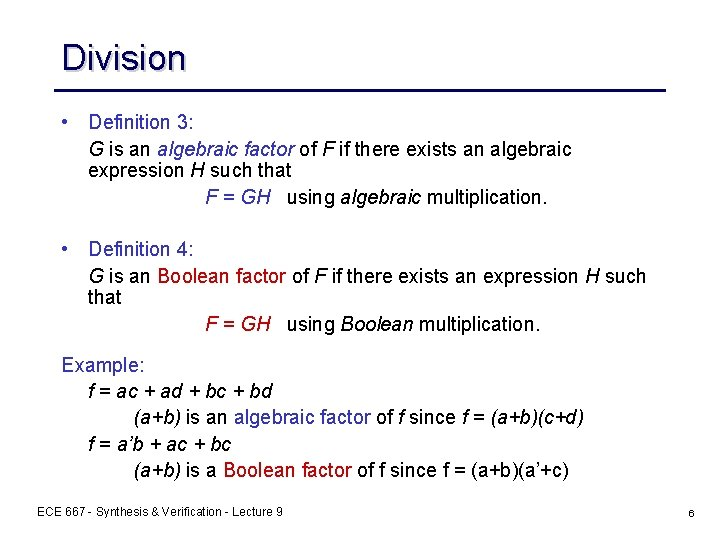Division • Definition 3: G is an algebraic factor of F if there exists an algebraic expression H such that F = GH using algebraic multiplication. • Definition 4: G is an Boolean factor of F if there exists an expression H such that F = GH using Boolean multiplication. Example: f = ac + ad + bc + bd (a+b) is an algebraic factor of f since f = (a+b)(c+d) f = a’b + ac + bc (a+b) is a Boolean factor of f since f = (a+b)(a’+c) ECE 667 - Synthesis & Verification - Lecture 9 6Why Use Algebraic Methods? • Need spectrum of operations – algebraic methods provide fast algorithms • Treat logic function like a polynomial – efficient data structures – fast methods for manipulation of polynomials available • Loss of optimality, but results are quite good • Can iterate and interleave with Boolean operations • In specific instances slight extensions available to include Boolean methods ECE 667 - Synthesis & Verification - Lecture 9 7Weak Division Weak division is a specific case of algebraic division. • Definition 5: Given two algebraic expressions F and G, a division is called weak division if • it is algebraic and • R has as few cubes as possible. The quotient H resulting from weak division is denoted by F/G. THEOREM: Given expressions F and G, expressions H and R generated by weak division are unique. ECE 667 - Synthesis & Verification - Lecture 9 8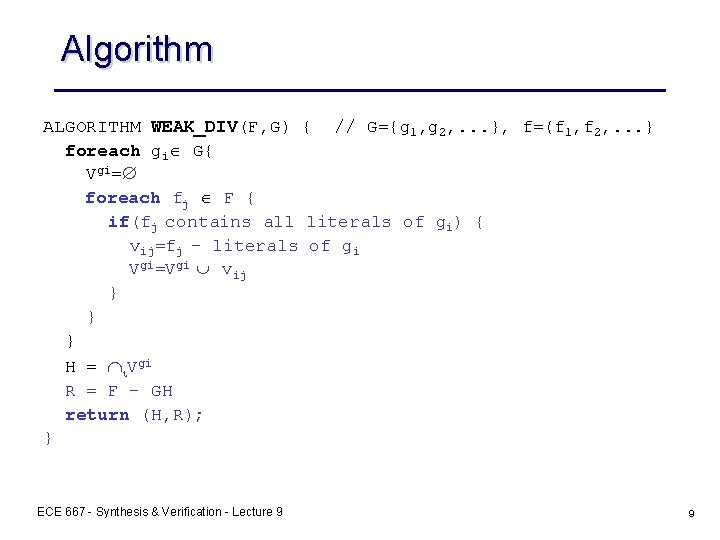Algorithm ALGORITHM WEAK_DIV(F, G) { // G={g 1, g 2, . . . }, f=(f 1, f 2, . . . } foreach gi G{ Vgi=Æ foreach fj F { if(fj contains all literals of gi) { vij=fj - literals of gi Vgi=Vgi È vij } } } H = Çi. Vgi R = F - GH return (H, R); } ECE 667 - Synthesis & Verification - Lecture 9 9Example of WEAK_DIV Example: divide F by G F = ace + ade + bc + bd + be +a’b + ab G = ae + b Vae= c + d Vb = c + d + e + a’ + a H = c + d = F/G H = Vg i R = be + a’b + ab R = F GH F = (ae + b)(c + d) + be + a’b + ab ECE 667 - Synthesis & Verification - Lecture 9 10Efficiency Issues We use filters to prevent trying a division. G is not an algebraic divisor of F if: • G contains a literal not in F. • G has more terms than F. • For any literal, its count in G exceeds that in F. • F is in the transitive fanin of G. ECE 667 - Synthesis & Verification - Lecture 9 11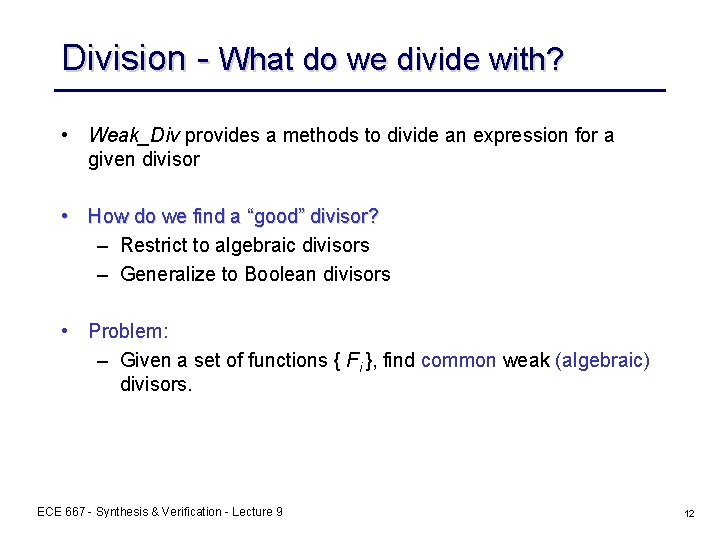Division - What do we divide with? • Weak_Div provides a methods to divide an expression for a given divisor • How do we find a “good” divisor? – Restrict to algebraic divisors – Generalize to Boolean divisors • Problem: – Given a set of functions { Fi }, find common weak (algebraic) divisors. ECE 667 - Synthesis & Verification - Lecture 9 12Kernels and Kernel Intersections • Definition 6: An expression is cube-free if no cube divides the expression evenly (i. e. there is no literal that is common to all the cubes). (ab + c) is cube-free (ab + ac) and abc are not cube-free Note: a cube-free expression must have more than one cube. • Definition 7: The primary divisors of an expression F are the set of expressions D(F) = { F/c | c is a cube }. ECE 667 - Synthesis & Verification - Lecture 9 13Kernels and Kernel Intersections • Definition 8: The kernels of an expression F are the set of expressions K(F) = {G | G D(F) and G is cube-free}. In other words, the kernels of an expression F are the cube-free primary divisors of F. • Definition 9: A cube c used to obtain the kernel K = F/c is called a co-kernel of K. C(F) is used to denote the set of co-kernels of F. ECE 667 - Synthesis & Verification - Lecture 9 14Example: x = adf + aef + bdf + bef + cdf + cef + g = (a + b + c)(d + e)f + g kernels co-kernels a+b+c d+e (a+b+c)(d+e)f+g df, ef af, bf, cf 1 ECE 667 - Synthesis & Verification - Lecture 9 15Fundamental Theorem THEOREM: If two expressions F and G have the property that k. F K(F), k. G K(G) | k. G k. F | 1 (i. e. , k. G and k. F have at most one term in common), then F and G have no common algebraic multiple divisors (i. e. with more than one cube). Important: If we “kernel” all functions and there are no nontrivial intersections, then the only common algebraic divisors left are single cube divisors. ECE 667 - Synthesis & Verification - Lecture 9 16The Level of a Kernel • Definition 10: A kernel is of level 0 (K 0) if it contains no kernels except itself. A kernel is of level n (Kn) if it contains at least one kernel of level (n-1), but no kernels (except itself) of level n or greater • K 0(F) K 1(F) K 2(F) . . . Kn(F) K(F). • level-n kernels = Kn(F) Kn-1(F) • Kn(F) is the set of kernels of level k or less. Example: F = (a + b(c + d))(e + g) k 1 = a + b(c + d) K 1 K 0 ==> level-1 k 2 = c + d k 3 = e + g ECE 667 - Synthesis & Verification - Lecture 9 K 0 17Kerneling Algorithm i = index of lexicographically ordered literals Algorithm KERNEL(j, G) { // li = literal associated with variable i R = Æ if(CUBE_FREE(G)) R = {G} // scan variables in predefined order // i = index of lexicographically ordered literals for(i=j+1, . . . , n) { if(li appears only in one term) continue if( k i, lk all cubes of G/li) continue R = R È KERNEL(i, MAKE_CUBE_FREE(G/li) } return R } MAKE_CUBE_FREE(F) removes algebraic cube factor from F ECE 667 - Synthesis & Verification - Lecture 9 18Kernel Generation - Example F = ace + bce + de + g // n = 6 variables, {a, b, c, d, e, g} • Call KERNEL (0, F) – i = 1, I 1 = a literal a appears only once, continue – i = 2, I 2 = b literal b appears only once, continue – i = 3, I 3 = c • make_cube_free(F/c) = (a+b) • call KERNEL(3, (a+b)) – the call considers variables 4, 5, 6 = {d, e, g} – no kernels • return R = {(a+b)} – i = 4, I 4 = d literal d appears only once, continue – i = 5, I 5 = e • make_cube_free(F/e) = (ac+bc+d) • call KERNEL(5, (ac+bc+d)) – the call considers variable 6 = {g} – no kernels – return R = {(a+b), (ac+bc+d)} – i = 6, I 6 = g literal g appears only once, continue, stop • Return R È {(a+b), (ac+bc+d), (ace+bce+de+g)} ECE 667 - Synthesis & Verification - Lecture 9 19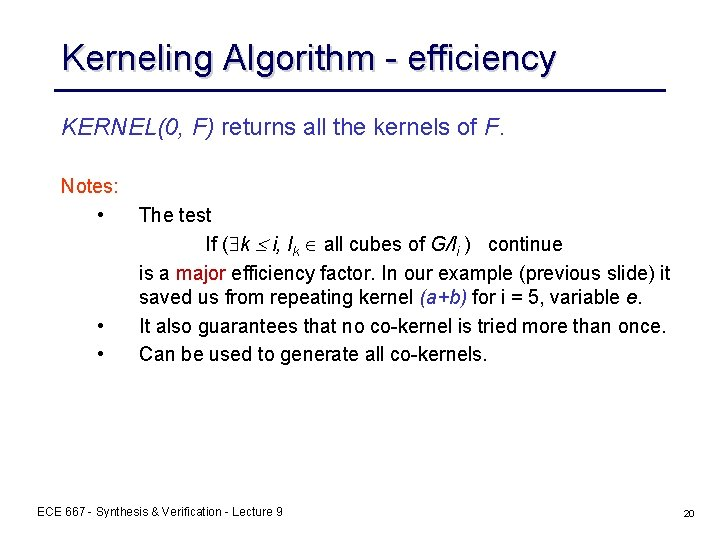Kerneling Algorithm - efficiency KERNEL(0, F) returns all the kernels of F. Notes: • • • The test If ( k i, lk all cubes of G/li ) continue is a major efficiency factor. In our example (previous slide) it saved us from repeating kernel (a+b) for i = 5, variable e. It also guarantees that no co-kernel is tried more than once. Can be used to generate all co-kernels. ECE 667 - Synthesis & Verification - Lecture 9 20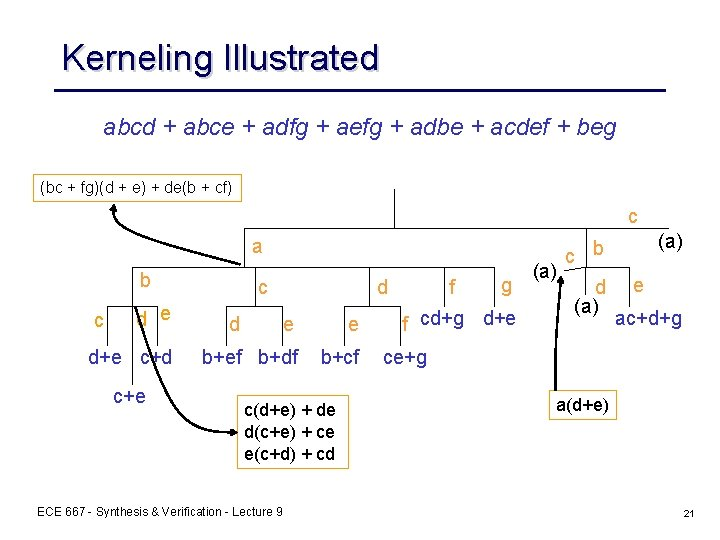Kerneling Illustrated abcd + abce + adfg + aefg + adbe + acdef + beg (bc + fg)(d + e) + de(b + cf) c a b c d e d+e c+d c+e c d d e e b+ef b+df b+cf c(d+e) + de d(c+e) + ce e(c+d) + cd ECE 667 - Synthesis & Verification - Lecture 9 f g f cd+g d+e (a) c b d (a) e ac+d+g ce+g a(d+e) 21Kerneling Illustrated co-kernels 1 a ab abc abd abe ac acd kernels a((bc + fg)(d + e) + de(b + cf))) + beg (bc + fg)(d + e) + de(b + cf) c(d+e) + de d+e c+d b(d + e) + def b + ef Note: f/bc = ad + ae = a(d + e) ECE 667 - Synthesis & Verification - Lecture 9 22Applications - Factoring Algorithm FACTOR(F) { if(F has no factor) return F // e. g. if |F|=1, or F is an OR of single literals // or of no literal appears more than once D = CHOOSE_DIVISOR(F) (Q, R) = DIVIDE(F, D) return FACTOR(Q)×FACTOR(D) + FACTOR(R) // recur } – – Different heuristics can be applied for CHOOSE_DIVISOR Different DIVIDE routines may be applied (e. g. also Boolean divide) ECE 667 - Synthesis & Verification - Lecture 9 23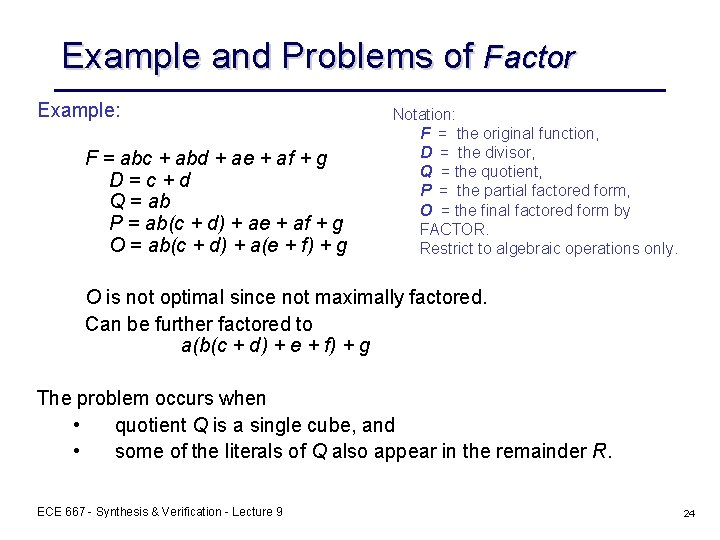Example and Problems of Factor Example: F = abc + abd + ae + af + g D=c+d Q = ab P = ab(c + d) + ae + af + g O = ab(c + d) + a(e + f) + g Notation: F = the original function, D = the divisor, Q = the quotient, P = the partial factored form, O = the final factored form by FACTOR. Restrict to algebraic operations only. O is not optimal since not maximally factored. Can be further factored to a(b(c + d) + e + f) + g The problem occurs when • quotient Q is a single cube, and • some of the literals of Q also appear in the remainder R. ECE 667 - Synthesis & Verification - Lecture 9 24Solving the Problem Solving this problem: • Check if the quotient Q is not a single cube, then done, else, • Pick a literal l 1 in Q which occurs most frequently in cubes of F. • Divide F by l 1 to obtain a new divisor D 1. Now, F has a new partial factored form (l 1)(D 1) + (R 1) and literal l 1 does not appear in R 1. Note: The new divisor D 1 contains the original D as a divisor because l 1 is a literal of Q. When recursively factoring D 1, D can be discovered again. ECE 667 - Synthesis & Verification - Lecture 9 25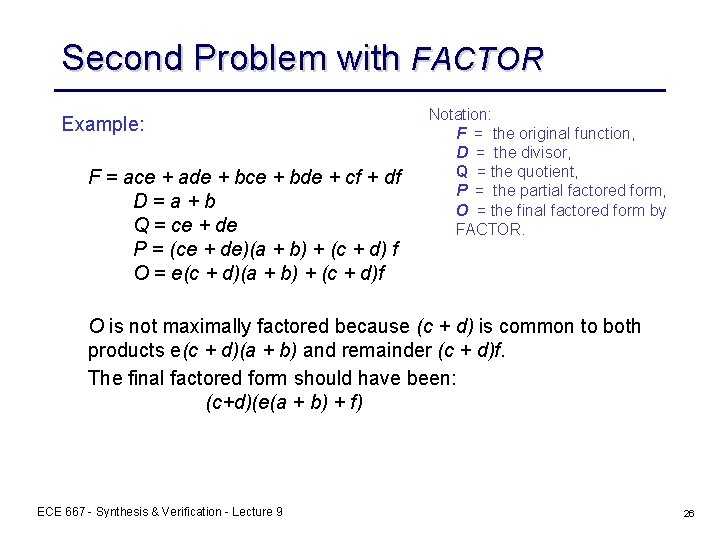Second Problem with FACTOR Example: F = ace + ade + bce + bde + cf + df D=a+b Q = ce + de P = (ce + de)(a + b) + (c + d) f O = e(c + d)(a + b) + (c + d)f Notation: F = the original function, D = the divisor, Q = the quotient, P = the partial factored form, O = the final factored form by FACTOR. O is not maximally factored because (c + d) is common to both products e(c + d)(a + b) and remainder (c + d)f. The final factored form should have been: (c+d)(e(a + b) + f) ECE 667 - Synthesis & Verification - Lecture 9 26Second Problem with FACTOR Solving the problem: • Essentially, we reverse D and Q ! • Make Q cube-free to get Q 1 • Obtain a new divisor D 1 by dividing F by Q 1 • If D 1 is cube-free, the partial factored form is F = (Q 1)(D 1) + R 1, and can recursively factor Q 1, D 1, and R 1 • If D 1 is not cube-free, let D 1 = c. D 2 and D 3 = Q 1 D 2. We have the partial factoring F = c. D 3 + R 1. Now recursively factor D 3 and R 1. ECE 667 - Synthesis & Verification - Lecture 9 27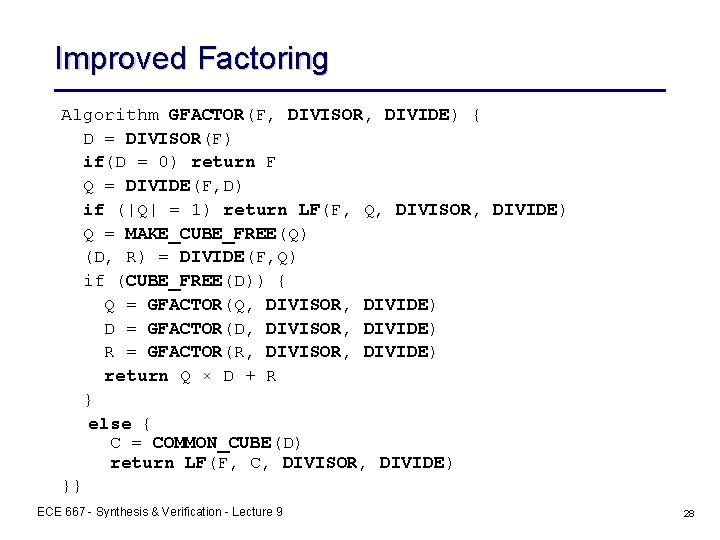Improved Factoring Algorithm GFACTOR(F, DIVISOR, DIVIDE) { D = DIVISOR(F) if(D = 0) return F Q = DIVIDE(F, D) if (|Q| = 1) return LF(F, Q, DIVISOR, DIVIDE) Q = MAKE_CUBE_FREE(Q) (D, R) = DIVIDE(F, Q) if (CUBE_FREE(D)) { Q = GFACTOR(Q, DIVISOR, DIVIDE) D = GFACTOR(D, DIVISOR, DIVIDE) R = GFACTOR(R, DIVISOR, DIVIDE) return Q × D + R } else { C = COMMON_CUBE(D) return LF(F, C, DIVISOR, DIVIDE) }} ECE 667 - Synthesis & Verification - Lecture 9 28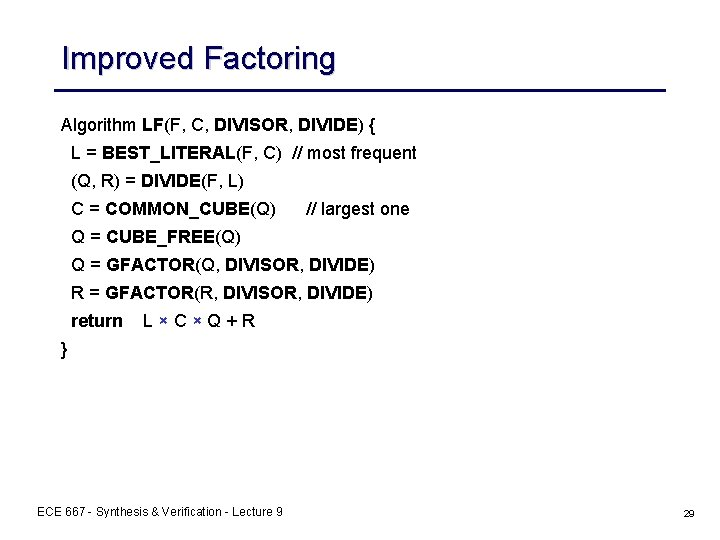Improved Factoring Algorithm LF(F, C, DIVISOR, DIVIDE) { L = BEST_LITERAL(F, C) // most frequent (Q, R) = DIVIDE(F, L) C = COMMON_CUBE(Q) // largest one Q = CUBE_FREE(Q) Q = GFACTOR(Q, DIVISOR, DIVIDE) R = GFACTOR(R, DIVISOR, DIVIDE) return L×C×Q+R } ECE 667 - Synthesis & Verification - Lecture 9 29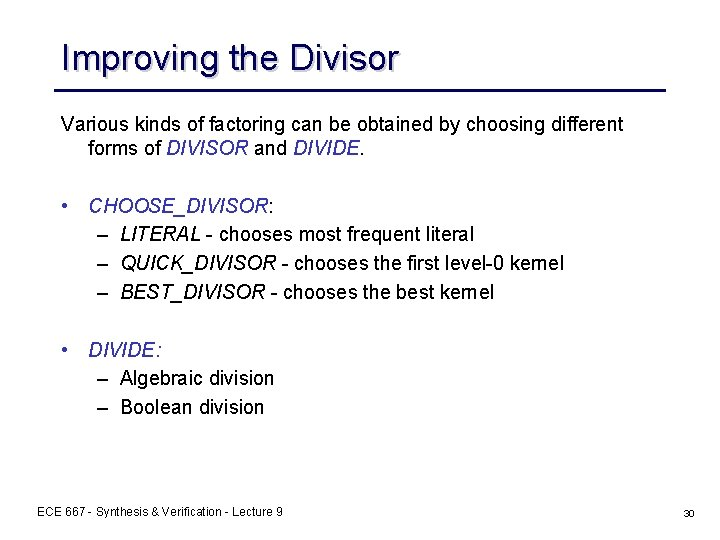Improving the Divisor Various kinds of factoring can be obtained by choosing different forms of DIVISOR and DIVIDE. • CHOOSE_DIVISOR: – LITERAL - chooses most frequent literal – QUICK_DIVISOR - chooses the first level-0 kernel – BEST_DIVISOR - chooses the best kernel • DIVIDE: – Algebraic division – Boolean division ECE 667 - Synthesis & Verification - Lecture 9 30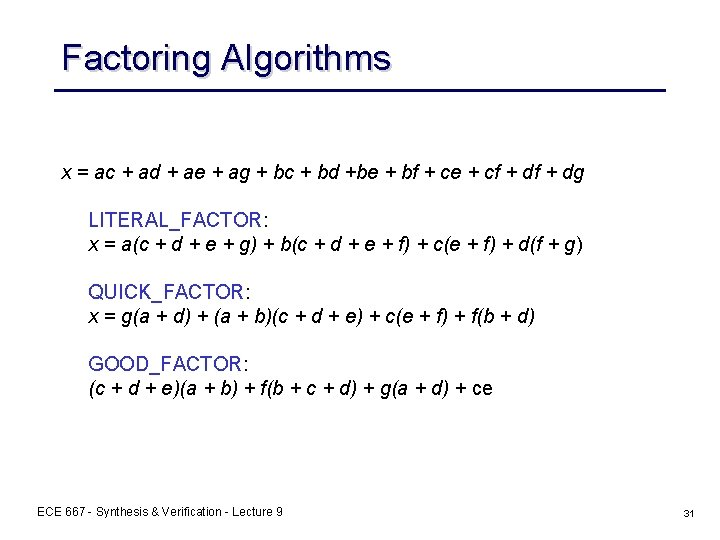Factoring Algorithms x = ac + ad + ae + ag + bc + bd +be + bf + ce + cf + dg LITERAL_FACTOR: x = a(c + d + e + g) + b(c + d + e + f) + c(e + f) + d(f + g) QUICK_FACTOR: x = g(a + d) + (a + b)(c + d + e) + c(e + f) + f(b + d) GOOD_FACTOR: (c + d + e)(a + b) + f(b + c + d) + g(a + d) + ce ECE 667 - Synthesis & Verification - Lecture 9 31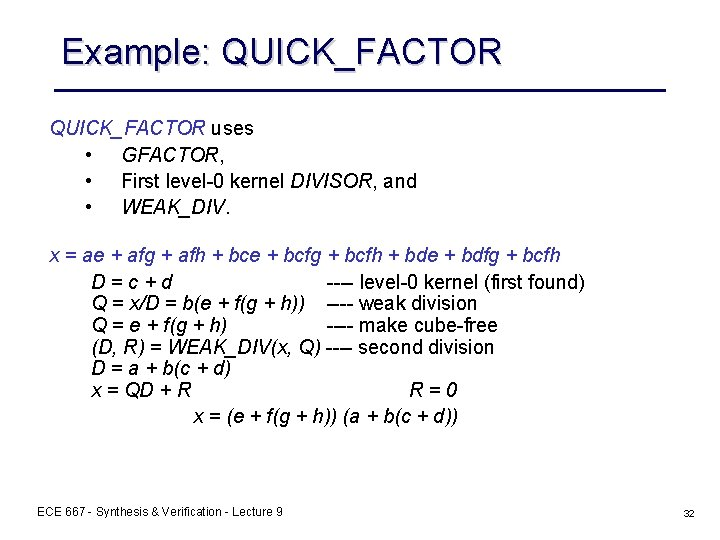Example: QUICK_FACTOR uses • GFACTOR, • First level-0 kernel DIVISOR, and • WEAK_DIV. x = ae + afg + afh + bce + bcfg + bcfh + bde + bdfg + bcfh D=c+d ---- level-0 kernel (first found) Q = x/D = b(e + f(g + h)) ---- weak division Q = e + f(g + h) ---- make cube-free (D, R) = WEAK_DIV(x, Q) ---- second division D = a + b(c + d) x = QD + R R=0 x = (e + f(g + h)) (a + b(c + d)) ECE 667 - Synthesis & Verification - Lecture 9 32Application - Decomposition is the same as factoring except: – divisors are added as new nodes in the network. – the new nodes may fan out elsewhere in the network in both positive and negative phases Algorithm DECOMP(fi) { k = CHOOSE_KERNEL(fi) if (k == 0) return fm+j = k // create new node m + j fi = (fi/k)ym+j+(fi/k’)y’m+j+r // change node i using new // node for kernel DECOMP(fi) DECOMP(fm+j) } Similar to factoring, we can define – – QUICK_DECOMP: pick a level 0 kernel and improve it. GOOD_DECOMP: pick the best kernel. ECE 667 - Synthesis & Verification - Lecture 9 33Re-substitution yj fi fj Idea: An existing node in a network may be a useful divisor in another node. If so, no loss in using it (unless delay is a factor). • Algebraic substitution consists of the process of algebraically dividing the function fi at node i in the network by the function fj (or by f’j) at node j. During substitution, if fj is an algebraic divisor of fj, then fi is transformed into fi = qyj + r • (or fi = q 1 yj + q 0 y’j + r ) In practice, this is tried for each node pair of the network. For n nodes in the network O(n 2) divisions. ECE 667 - Synthesis & Verification - Lecture 9 34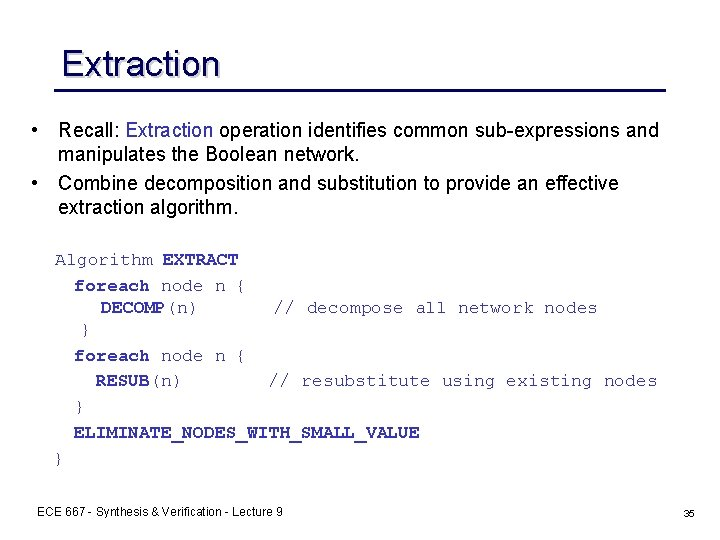Extraction • Recall: Extraction operation identifies common sub-expressions and manipulates the Boolean network. • Combine decomposition and substitution to provide an effective extraction algorithm. Algorithm EXTRACT foreach node n { DECOMP(n) // decompose all network nodes } foreach node n { RESUB(n) // resubstitute using existing nodes } ELIMINATE_NODES_WITH_SMALL_VALUE } ECE 667 - Synthesis & Verification - Lecture 9 35Extraction Kernel Extraction: 1. Find all kernels of all functions 2. Choose kernel intersection with best “value” 3. Create new node with this as function 4. Algebraically substitute new node everywhere 5. Repeat 1, 2, 3, 4 until best value threshold New Node ECE 667 - Synthesis & Verification - Lecture 9 36Example-Extraction f 1 = ab(c(d + e) + f + g) + h, f 2 = ai(c(d + e) + f + j) + k (only level-0 kernels used in this example) 1. Extraction: l=d+e K 0(f 1) = K 0(f 2) = {d + e} K 0(f 1) K 0(f 2) = {d + e} f 1 = ab(cl + f + g) + h f 2 = ai(cl + f + j) + k 2. Extraction: K 0(f 1) = {cl + f + g}; K 0(f 2) = {cl + f + j) K 0(f 1) K 0(f 2) = cl + f m = cl + f f 1 = ab(m + g) + h f 2 = ai(m + j) + k No kernel intersections anymore ! 3. Cube extraction: n = am f 1 = b(n + ag) + h f 2 = i(n + aj) + k ECE 667 - Synthesis & Verification - Lecture 9 37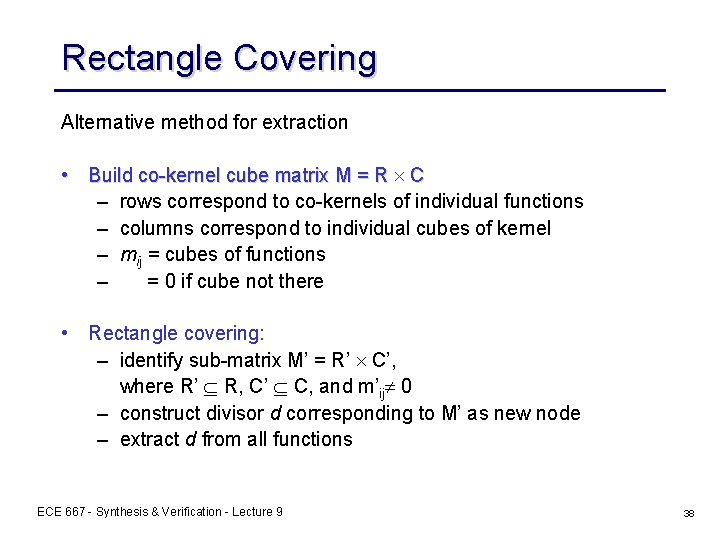Rectangle Covering Alternative method for extraction • Build co-kernel cube matrix M = R ´ C – rows correspond to co-kernels of individual functions – columns correspond to individual cubes of kernel – mij = cubes of functions – = 0 if cube not there • Rectangle covering: – identify sub-matrix M’ = R’ ´ C’, where R’ Í R, C’ Í C, and m’ij¹ 0 – construct divisor d corresponding to M’ as new node – extract d from all functions ECE 667 - Synthesis & Verification - Lecture 9 38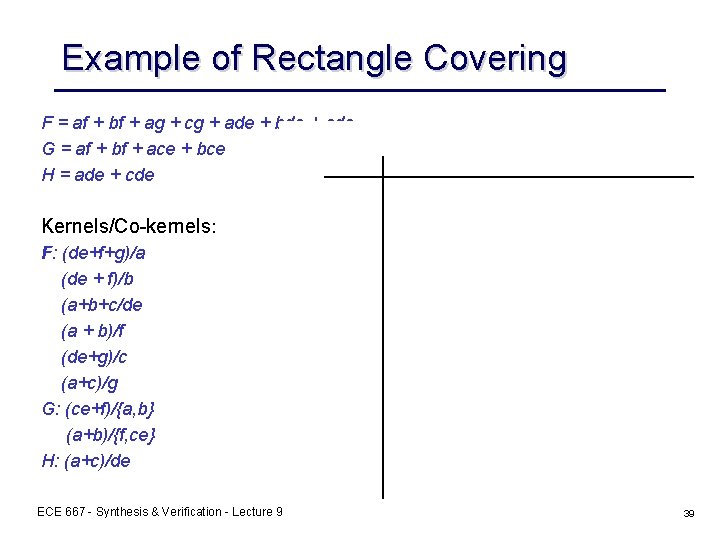Example of Rectangle Covering F = af + bf + ag + cg + ade + bde + cde G = af + bf + ace + bce H = ade + cde Kernels/Co-kernels: F: (de+f+g)/a (de + f)/b (a+b+c/de (a + b)/f (de+g)/c (a+c)/g G: (ce+f)/{a, b} (a+b)/{f, ce} H: (a+c)/de ECE 667 - Synthesis & Verification - Lecture 9 39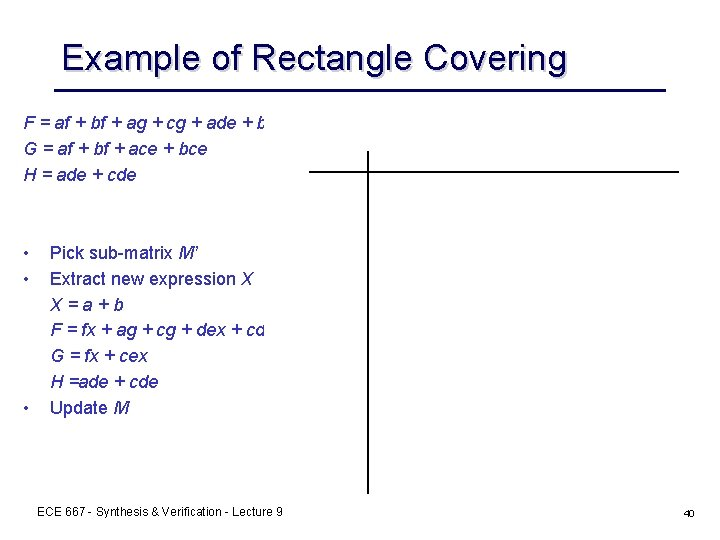Example of Rectangle Covering F = af + bf + ag + cg + ade + bde + cde G = af + bf + ace + bce H = ade + cde • • • Pick sub-matrix M’ Extract new expression X X=a+b F = fx + ag + cg + dex + cde G = fx + cex H =ade + cde Update M ECE 667 - Synthesis & Verification - Lecture 9 40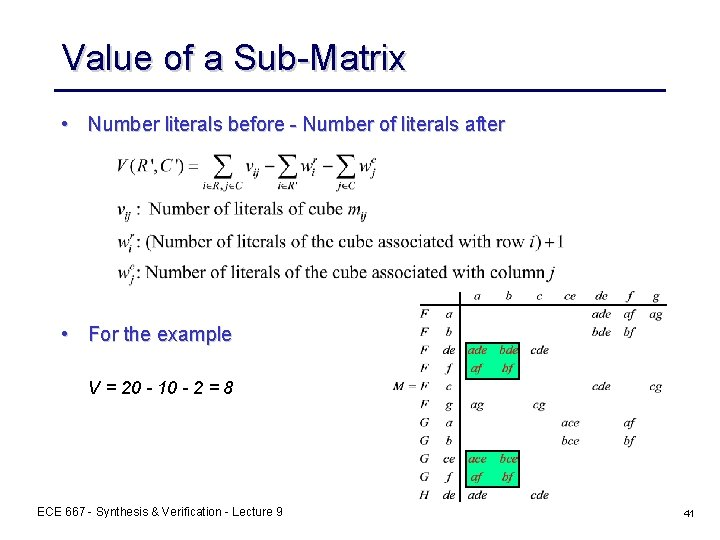Value of a Sub-Matrix • Number literals before - Number of literals after • For the example V = 20 - 10 - 2 = 8 ECE 667 - Synthesis & Verification - Lecture 9 41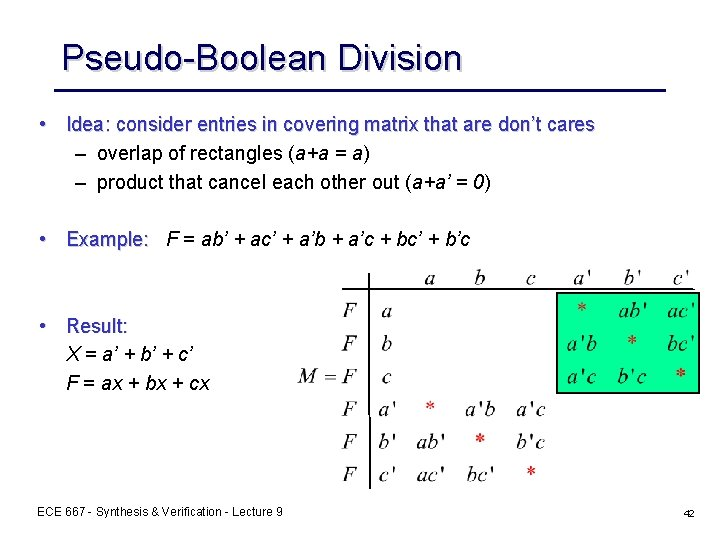Pseudo-Boolean Division • Idea: consider entries in covering matrix that are don’t cares – overlap of rectangles (a+a = a) – product that cancel each other out (a+a’ = 0) • Example: F = ab’ + ac’ + a’b + a’c + bc’ + b’c • Result: X = a’ + b’ + c’ F = ax + bx + cx ECE 667 - Synthesis & Verification - Lecture 9 42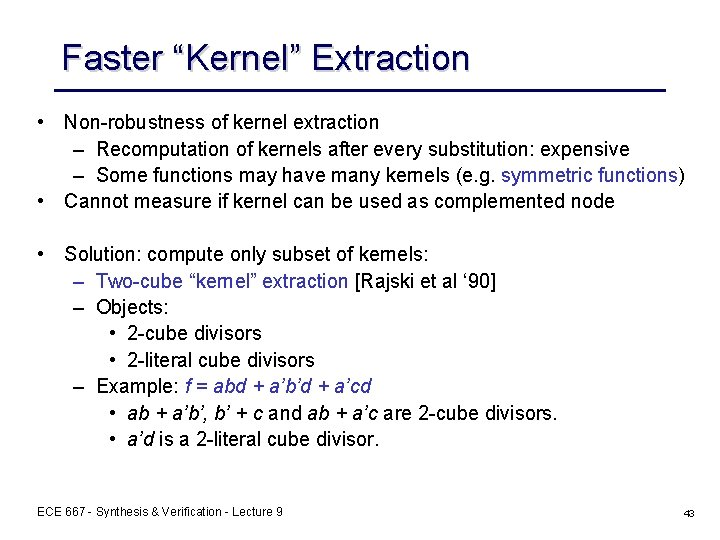Faster “Kernel” Extraction • Non-robustness of kernel extraction – Recomputation of kernels after every substitution: expensive – Some functions may have many kernels (e. g. symmetric functions) • Cannot measure if kernel can be used as complemented node • Solution: compute only subset of kernels: – Two-cube “kernel” extraction [Rajski et al ‘ 90] – Objects: • 2 -cube divisors • 2 -literal cube divisors – Example: f = abd + a’b’d + a’cd • ab + a’b’, b’ + c and ab + a’c are 2 -cube divisors. • a’d is a 2 -literal cube divisor. ECE 667 - Synthesis & Verification - Lecture 9 43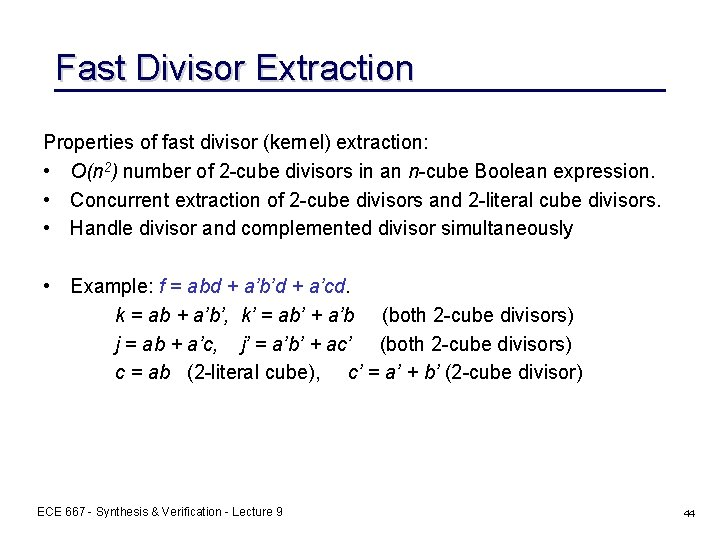Fast Divisor Extraction Properties of fast divisor (kernel) extraction: • O(n 2) number of 2 -cube divisors in an n-cube Boolean expression. • Concurrent extraction of 2 -cube divisors and 2 -literal cube divisors. • Handle divisor and complemented divisor simultaneously • Example: f = abd + a’b’d + a’cd. k = ab + a’b’, k’ = ab’ + a’b (both 2 -cube divisors) j = ab + a’c, j’ = a’b’ + ac’ (both 2 -cube divisors) c = ab (2 -literal cube), c’ = a’ + b’ (2 -cube divisor) ECE 667 - Synthesis & Verification - Lecture 9 44Generating All 2 -cube Divisors F = {ci}, D(F) = {d | d = make_cube_free(ci + cj)} This takes all pairs of cubes in F and makes them cube-free. ci, cj are any pair of cubes in F Divisor generation is O(n 2), where n = number of cubes in F Example: F = axe + ag + bcxe + bcg make_cube_free(ci + cj) = {xe + g, a + bc, axe + bcg, ag + bcxe} Note: • the function F is made into an algebraic expression before generating double-cube divisors • not all 2 -cube divisors are kernels (why ? ) ECE 667 - Synthesis & Verification - Lecture 9 45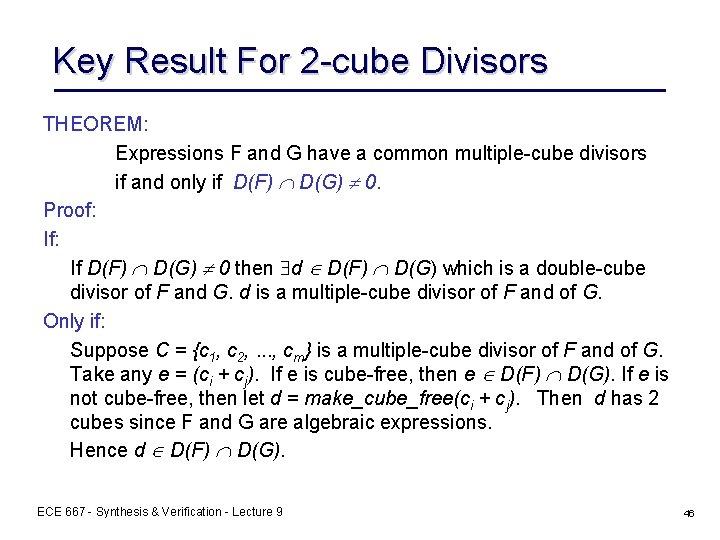Key Result For 2 -cube Divisors THEOREM: Expressions F and G have a common multiple-cube divisors if and only if D(F) D(G) 0. Proof: If D(F) D(G) 0 then d D(F) D(G) which is a double-cube divisor of F and G. d is a multiple-cube divisor of F and of G. Only if: Suppose C = {c 1, c 2, . . . , cm} is a multiple-cube divisor of F and of G. Take any e = (ci + cj). If e is cube-free, then e D(F) D(G). If e is not cube-free, then let d = make_cube_free(ci + cj). Then d has 2 cubes since F and G are algebraic expressions. Hence d D(F) D(G). ECE 667 - Synthesis & Verification - Lecture 9 46Key Result For 2 -cube Divisors Example: Suppose that C = ab + ac + f is a multiple divisor of F and G. If e = ac + f, e is cube-free and e D(F) D(G). If e = ab + ac, d = {b + c} D(F) D(G) As a result of the Theorem, all multiple-cube divisors can be “discovered” by using just double-cube divisors. ECE 667 - Synthesis & Verification - Lecture 9 47Fast Divisor Extraction Algorithm: • Generate and store all 2 -cube kernels (2 -literal cubes) and recognize complement divisors. • Find the best 2 -cube kernel or 2 -literal cube divisor at each stage and extract it. • Update 2 -cube divisor (2 -literal cubes) set after extraction • Iteratate extraction of divisors until no more improvement • Results: – Much faster – Quality as good as that of kernel extraction ECE 667 - Synthesis & Verification - Lecture 9 48